There are all sorts of resonances around us, in the world, in our culture, and in our technology. A tidal resonance causes the 55 foot tides in the Bay of Fundy. Mechanical and acoustical resonances and their control are at the center of practically every musical instrument that ever existed. Even our voices and speech are based on controlling the resonances in our throat and mouth. Technology is also a heavy user of resonance. All clocks, radios, televisions, and gps navigating systems use electronic resonators at their very core. Doctors use magnetic resonance imaging or MRI to sense the resonances in atomic nuclei to map the insides of their patients. In spite of the great diversity of resonators, they all share many common properties. In this blog, we will delve into their various aspects. It is hoped that this will serve both the students and professionals who would like to understand more about resonators. I hope all will enjoy the animations.

Origins of Newton's laws of motion

History of mechanical clocks with animations
Understanding a mechanical clock with animations
includes pendulum, balance wheel, and quartz clocks

## Detailed conformal mappingsBelow in Fig. 26, we present detailed conformal mappings using a variety of analytic functions.

On each mapping, we label and number both the u-v axes (in black) and the x-y axes (in beige). You may need to look carefully for the x-y labels and number ... they are a little hard to make out in places. The grids for the u-v coordinates are shown as dotted lines, while the grids for the x-y coordinates are shown in beige.

There is a color overlay, with a legend, on the mappings showing the magnitude of the gradient as it varies across each mapping. This is calculated using the formula |dz/dw|. It turns out that this one formula is valid for all the mappings, independent of whether the initial equipotential lines are horizontal or vertical, and independent of their direction of increase. Thus if you are using the mapping in Fig. 26a to understand fluid flow through a corner, then the magnitude of the velocity of the fluid (which equals minus the gradient) is given by the color map for either flow from the left or flow to the left. Likewise, if you are using this mapping to understand electric fields around a charged metal angle, the color map gives the electric field magnitude. We see that in either the fluid case or the electric field case, the corner represents a dead area in which the gradient goes to zero.

At the bottom, after the mappings, we discuss shifting and scaling, which allows these maps to be shifted and stretched to serve a larger variety of applications.w = z0.5 Fig. 26a. Mapping by the function   w = z0.5   where the domain of   z = x + iy   is   −2 ≤ x ≤ 2   and   0 ≤ y ≤ 2  . Note that the very corner (next to the origin) is a "dead" zone with a gradient of almost zero (no flow in the fluid case). This mapping could be used for the electric field around a charged metal angle or the fluid flow field being diverted by such an angle. See our first and fourth postings on conformal mapping for more.w = z1.5 Fig. 26b. Mapping by the function   w = z1.5   where the domain is   −2 ≤ x ≤ 2   and   0 ≤ y ≤ 2  . In this case the corner is a very active region where the maximum gradient occurs. In fact the gradient tends to infinity as we approach the exact corner. This mapping could be used for the electric field near a charged metal angle or fluid flow field around a corner. See the link in Fig. 26a for more on applications.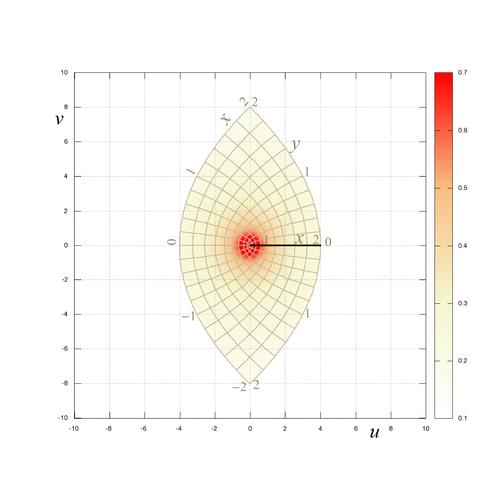w = z2 Fig. 26b'. Mapping by the function   w = z2   where the domain is   −2 ≤ x ≤ 2   and   0 ≤ y ≤ 2  . The corner is again the active region where the maximum gradient occurs, actually going to infinity as in the above mapping. The black line indicates a break where the negative x-axis is warped around to be on top of the positive x-axis. This mapping could be used for the electric field near the edge of charged metal plate or fluid flow field around the edge of a plate. See the link in Fig. 26a for more on applications.w = ez Fig. 26c. Mapping by the function   w = ez   where the domain is   0.1 ≤ x ≤ 1   and   0 ≤ y ≤ 3.1  . If we had extended y to include π our figure would have been a complete semicircle, or extended to 2π it would have been a circle. The gradient is inversely proportional to the polar radius, (u2 + v2)0.5  and therefore larger nearer the origin. This mapping could be used for the electric, magnetic, or fluid flow field around a long cylindrical source. See the third and fourth conformal mapping postings for more on these applications.w = sin z Fig. 26d. Mapping by the function  w = sin z   where the domain is   −1.5 ≤ x ≤ 3.1   and   0 ≤ y ≤ 1  . We extended the domain of x to be larger than π/2 to illustrate how this mapping function wraps the original grid around the point (1,0) into the region below the x-axis. The dark black line indicates a discontinuity at the x-axis between u = −1 and u = 1. This mapping could represent flow going around a metal plate. See the third and fourth postings for more on applications of this mapping. The gradient tends to infinity as one approaches either of the points (u,v) = (−1,0) or (1,0) .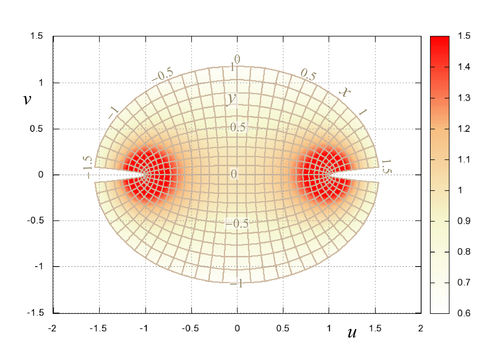w = sinz Fig. 26e. Second mapping by the function w = sin z   with a different domain. The domain is now  −1.5 ≤ x ≤ 1.5  and  −1 ≤ y ≤ 1 . This mapping illustrates extending the domain into the negative y space and restricting the x space to less than  ±π/2 . As above,the gradient tends to infinity as one approaches either of the points (u,v) = (−1,0) or (1,0) . This mapping could be used to model fluid flow through a slit or the electric fields between two charged metal plates. See the link for Fig. 26d for details. This mapping also shows the fields around a slotline type transmission line (if we ignore the dielectric-air interface) used in microwave communication devices.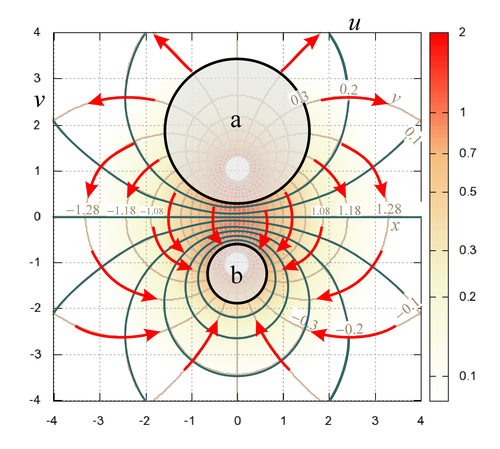w = tan z Fig. 26f. Mapping by the function   w = tanz  . The domain is  −1.5 ≤ x ≤ 1.5  and  −1 ≤ y ≤ 1 . As above,the gradient tends to infinity as one approaches either of the points (u,v) = (0,1) or (0,−1) . This mapping could describe the electric field around two parallel conductors or the fluid flow between a source and sink dipole. It shows the electric fields around a two-wire transmission line (see most electromagnetics texts such as shown here pages 413-415 or Cheng, 2nd ed, pp 164+ (fields picture on page 167) ). The upper mapping is not extended into the top-center and bottom-center regions because one more increment of the grid would not fit in these places. The lower mapping uses the somewhat strange increment dx = π/32 to allow extending x all the way to ±π/2 and filling in of these regions. The lower mapping also uses a color log scale to better show the range of the gradient. In the lower mapping, we added vectors, colored lines, and circles to illustrate an important application of this mapping, to display the electric fields between two oppositely charged cylindrical conductors. The black circles, labeled a and b, show two possible locations of these conductors, held at two different electrical potentials. The grid lines that are between the two circles show the electric fields (with red vectors added) and electric equipotentials (in green). We ignore the grids inside the circles (thus they are whited out). In use, we look for the combination of possible circles on the mapping that match the same ratio of the separation distance-to-radii as the application at hand, then scale up the whole mapping as needed. We see from the color map, that the gradient, i.e. the electric field, is most intense in the gap between the cylinders and has very low values a distance away from the gap. This plot could also be used for the electric fields between two cylindrical metal tubes, one inside the other, and not concentrically positioned (concentric tubes can be handled by Fig. 26c, see Fig. 23b). For example, we might use circle "a" for one conductor and one of the circular grids that is inside "a" for the second conductor. The grids inside "a" and outside the second conductor are the electric field and equipotential lines. We can also use one circle and the straight horizontal line in the center to show the electric fields between a charged cylindrical conductor and grounded metal sheet.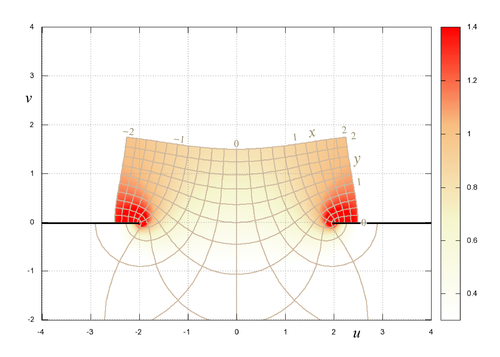w = z + 1/z Fig. 26g. Mapping by the function   w = z + 1/z   where the domain is   −2 ≤ x ≤ 2   and   0 < y ≤ 2  . The dark black lines indicate discontinuities for |u| > 2 . The gradient tends to infinity as we approach either point (u,v) = (−2,0) or (2,0) . This mapping could describe the electric field around a charged metal plate having a deep cavity in it, or the flow along the top of a similar plate (with a deep cavity in it). See the third and fourth postings on conformal mapping for more application discussion.w = 1/z Fig. 26h. Mapping by the function   w = 1/z   where the domain is   0.2 ≤ x ≤ 1   and   −1 < y ≤ 1  . The gradient tends to infinity as we approach the origin, i.e. the point (u,v) = (0,0) . This mapping describes the electric, magnetic, or fluid flow field around a tiny but strong dipole source (in two dimensions). See Wikipedia for more on dipole fields. This figure is strikingly similar to the Smith chart used to understand wave reflections and design microwave communication components, even down to the use of complex numbers. The Smith chart has nothing to do with Laplace's equation, but involves inverting complex numbers (with an offset).### Shifting and scaling

By shifting and scaling we can greatly extend the utility of the above mappings. Scaling consists of multiplying our starting potential or position coordinates (x,y) by a constant, i.e. (x,y) becomes (ax,ay) where a is a constant. Shifting consists of adding or subtracting a constant from the potentials or coordinates, i.e. (x,y) becomes (x + b,y + c) where b and c are constants. Both operations can be performed before and/or after the conformal mapping has been done.

Shifting before the transform coordinates would allow the plate and slot in Fig. 26g to be moved away from its position shown in that figure, while scaling would allow a change in the slot width.

The figures below illustrate the effects of shifting and scaling.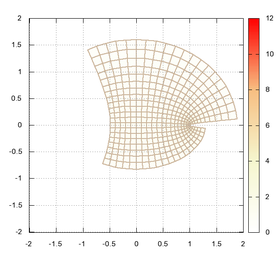Fig. 27a. Starting map of  w = sin z  with -1 ≤ x ≤ 1  and -1 ≤ y ≤ 1 . Fig. 27b. Pre-shifted (shifted before the sinz mapping) by the amount 0.5 + 0.25i , i.e. z → z + (1 + 0.25i) . If simply applied to a rectangular grid this only shifts the domain of the grid that the transform works on. If there is an initial starting potential, then this shifts that potential before the transform. Fig. 27c. Pre-scaled by 0.5, i.e. z → 0.5z . If simply applied to a rectangular grid this scales the domain of the grid that the transform works on. If there is an initial starting potential, then this scales that potential before the transform.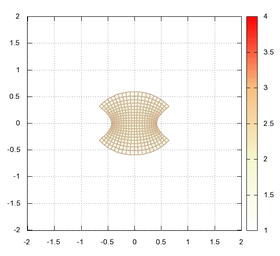Fig. 27d. Both pre-shifted and pre-scaled, i.e. z → 0.5[z + (1 + 0.25i)] . Fig. 27e. Post shifted by the amount 0.5 + 0.25i , i.e. w → w + (1 + 0.25i) . This shifts the final result of the transform. Fig. 27f. Post scaled by 0.5, i.e. w → 0.5w . This scales the final transform result to make it larger or smaller.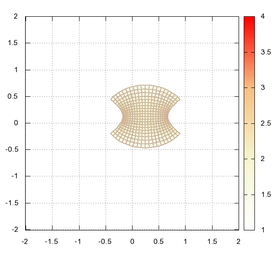Fig. 27g. Both post shifted and post scaled, i.e. w → 0.5[w + (1 + 0.25i)] . This both shifts the final result and shrinks or expands it.

Overview

• The above material on conformal mappings has been presented in less than rigorous fashion, stressing instead the visual nature of these mappings and their applications. In this final box, we summarize and add a little more mathematics to the study. More rigorous teachings are available in texts and on the internet (see the links below).

• A conformal transform (or mapping) maps the space z = (x,y) into another space w = (u,v), where each point in the z-space is mapped into a point in the w-space. In our postings we have illustrated many of these mappings, showing how an xy grid is mapped into a uv grid system.

• One of the properties of conformal mappings is that a solution to Laplace's equation in z space will be mapped by a conformal mapping to another solution to Laplace's equation in w space. That is to say, if  Φ0(x,y) = Φ0(z) is a solution to Laplace's equation in z space,, then the mapped solution Φ1(u,v) will also be a solution to Laplace's equation,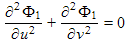. If  w = f(z) is our mapping function and  z = f −1(z)  is its inverse, then the mapped potential is given by:  Φ1(w) = Φ0(z)  = Φ0(f −1(w) ).

For example, suppose we let  w = f(z) = ez  be our mapping function, then  z = f −1(w) = logw  must be the inverse function. In addition, suppose we let our potential be given by the horizontal coordinate: Φ0(z) = x = Real(z) .
Putting this all together, we get Φ1(w) = Φ0(z) = Real(z) = Φ0(f −1(w) ) = Real(logw)  as our mapped potential. Fluid velocity or electric field would be given by negative the gradient of this mapped potential. We saw this math before in the caption of Fig. 9a of the third posting on conformal mapping.

• The most common starting solution is made by setting Φ0 equal the x or y value (or its negative) of our z coordinate system. That is: Φ0 = x  or  y  or  −x  or  −y , as we illustrated in the example immediately above and illustrated in Fig. 20 of the previous posting. This potential may be shifted and scaled, as desired, i.e. Φ0 = ax + b , where a and b are constants. We call this initial choice "the rectilinear starting grid".

• With this choice of starting solution, one set of grids become the equipotential lines and the other perpendicular grids become the streamlines (showing the direction of the gradient field, i.e. the fluid velocity direction or electric fields). This was illustrated in Fig. 20 of the previous posting.

• Because the potential along a single "equipotential" grid line is constant, any of the "equipotential" grid lines is a possible Dirichlet boundary, especially since shifting and scaling can be used to shift and scale the actual values of the potentials on these grid lines. In general, a Dirichlet boundary is an edge of our space of interest for which the potential Φ takes on a known, constant value, whereas a Neumann boundary is an edge that has a known gradient.

• In the study of fluid flow, and electric and magnetic fields, values of the gradient along a Neumann boundary are usually not known. One usually only knows the fact that the gradient points in the direction parallel to the boundary. This insures, for example, that fluid will not flow through impenetrable surfaces. Because all "gradient" grid lines run parallel to the gradient, we can use any of the "gradient" grid lines as a Neumann boundary without regard to the actual values of the gradients. Only the shape of a mapped "gradient" grid line is important.

• Although we did not discuss this above, multiple conformal mappings can be applied serially and still the mapped potentials will be solutions to Laplace's equation. In fact the scaling and shifting operations can be considered as conformal mappings (they are analytic functions) which allows them to be done before or after our main conformal mapping while maintaining our potential as a solution to Laplace's equation.

• Most of the references below show, in addition to mappings of the rectilinear grid, the result of mapping the polar coordinate system into another space. The polar coordinate system itself is the result of first using the transform  w = ez  on the rectilinear system, shown in Fig. 26c above and also in our third and fourth conformal mapping postings.

• While some of the above may seem very mathematical, in practice, using conformal mappings is very visual in nature. It involves picking a mapping function (from a library of functions) that looks about right and fits the geometry of the problem at hand. On the other hand, getting full use of the conformal mapping thus picked out, is enhanced by knowing the math involved.

1. An extensive library of conformal mappings by John Mathews and Russell Howell. See links to additional pages of mappings and material at the bottom of this web site.
2. Wikipedia on conformal mapping.
3. A beautiful set of conformal mappings.
4. Wolfram, an illustrated teaching on conformal mapping.
5. NASA. Conformal mappings applied to airplane wings.
6. An interactive conformal mapping demo. Be patient as it loads. Drag the array of green dots around the area of the screen to see how a particular mapping transforms the initial rectangular grid. Also click on the "Next Map" button for a few more mappings.
7. An ad for a book on conformal mappings. The ad itself has a few very interesting mappings, showing some of the more extreme possibilities.
8. An extensive list of more links and resources on the subject.Copyright, P. Ceperley, September, 2010.